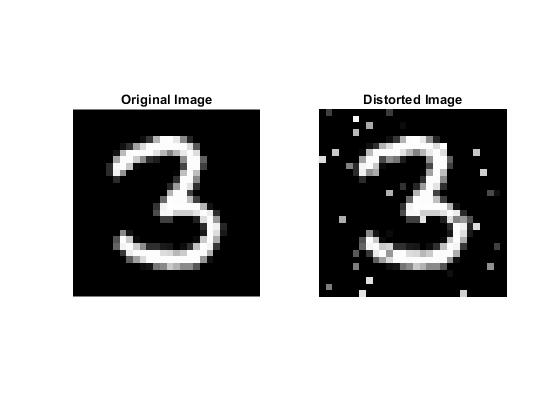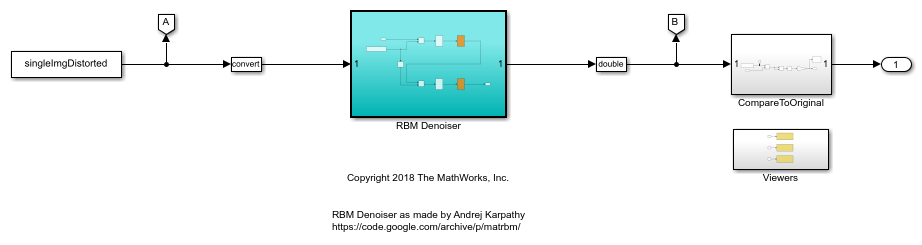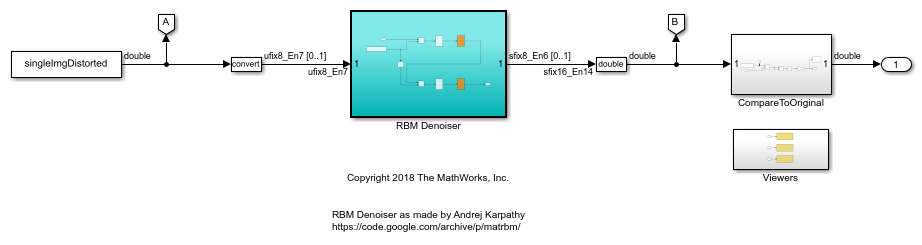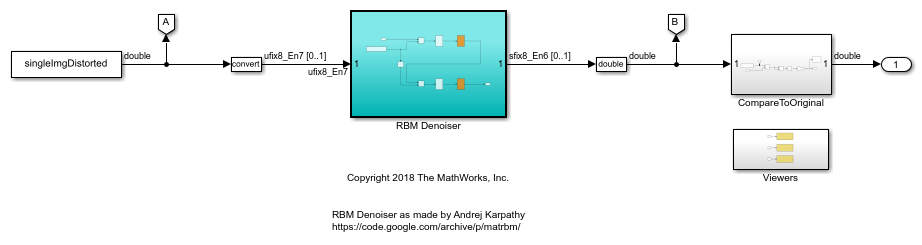# Image Denoising Using Fixed-Point Quantized Restricted Boltzmann Machine Algorithm

This example highlights two workflows that can help you arrive at a fully embedded-efficient fixed-point design. This example shows how to:

• Use multiple simulation scenarios in data type optimization.

• Use ranges derived from design ranges for data-type optimization.

• Use different benchmarks of numerical behavior for each scenario using blocks from the Model Verification library.

• Replace math operations that do not support fixed-point data types with efficient lookup tables.

### Convert Model to Use Optimal Fixed-Point Data Types

The model in this example uses a Restricted Boltzmann Machine (RBM) algorithm to denoise images. Load the image data and RBM algorithm weights. The original and distorted images are stored in the `imgOriginal` and `imgDistorted` variables. Each row of each matrix is a test image from the MNIST data set.

```load RBMData; ```

Open and view the first set of test images.

```singleImgDistorted = imgDistorted(1,:); singleImgOriginal = imgOriginal(1,:); imgSize = length(singleImgOriginal); subplot(1,2,1) imshow(reshape(singleImgOriginal,[28,28])') title('Original Image'); subplot(1,2,2) imshow(reshape(singleImgDistorted,[28,28])') title('Distorted Image') ```Open the model. The model loads a distorted test image, uses the RBM algorithm to denoise the image, and then compares the denoised image to the original image without added noise. To improve simulation speed, the video display is turned off in this model. To turn on the video display, set the `DISPLAY_VIEWER` variable to 1.

```model = 'ex_rbmDenoiser01'; open_system(model); DISPLAY_VIEWER = 0; ```When converting a model to use fixed-point data types, it is important to collect ranges while exercising the model over its full operating range. You can do this by defining multiple simulation scenarios. In this example, each of the five simulation scenarios defines a new set of test images to denoise and compare to the original image.

```IMGN = 5; si = Simulink.SimulationInput.empty(0, IMGN); for indx = 1:IMGN si(indx) = Simulink.SimulationInput(model); si(indx) = si(indx).setVariable('singleImgDistorted', imgDistorted(indx,:)); si(indx) = si(indx).setVariable('singleImgOriginal', imgOriginal(indx,:)); end ```

In each simulation scenario, verify that the mean-squared error between the original image and the denoised image is less than 0.02.

``` si(1) = si(1).setBlockParameter([model '/CompareToOriginal/check'], 'max', '0.02'); si(2) = si(2).setBlockParameter([model '/CompareToOriginal/check'], 'max', '0.02'); si(3) = si(3).setBlockParameter([model '/CompareToOriginal/check'], 'max', '0.02'); si(4) = si(4).setBlockParameter([model '/CompareToOriginal/check'], 'max', '0.02'); si(5) = si(5).setBlockParameter([model '/CompareToOriginal/check'], 'max', '0.03'); ```

Define the options to use during optimization. For this example, restrict the word lengths in the converted model to be between 8 and 16 bits. You can also restrict the number of iterations the optimization algorithm performs.

```options = fxpOptimizationOptions(... 'AllowableWordLengths', [8 16], ... 'MaxIterations', 50, ... 'Patience', 50); ```

To collect derived ranges in the model in addition to using the simulation scenarios to collect simulation ranges, set the `UseDerivedRangeAnalysis` option to `true`. Derived range analysis often returns a more conservative estimate of the dynamic ranges in the system than ranges collected through simulations.

```options.AdvancedOptions.UseDerivedRangeAnalysis = true; ```

Specify the simulation scenarios to use during the optimization.

```options.AdvancedOptions.SimulationScenarios = si; ```

Use the `fxpopt` function to optimize the data types in the RBM Denoiser subsystem according to the options specified in the `fxpOptimizationOptions` object, `options`

```result = fxpopt(model, [model '/RBM Denoiser'], options); ```
``` + Starting data type optimization... + Checking for unsupported constructs. + Preprocessing + Modeling the optimization problem - Constructing decision variables + Running the optimization solver - Evaluating new solution: cost 344, does not meet the behavioral constraints. - Evaluating new solution: cost 656, meets the behavioral constraints. - Updated best found solution, cost: 656 - Evaluating new solution: cost 640, meets the behavioral constraints. - Updated best found solution, cost: 640 - Evaluating new solution: cost 624, does not meet the behavioral constraints. - Evaluating new solution: cost 632, meets the behavioral constraints. - Updated best found solution, cost: 632 - Evaluating new solution: cost 608, meets the behavioral constraints. - Updated best found solution, cost: 608 - Evaluating new solution: cost 600, meets the behavioral constraints. - Updated best found solution, cost: 600 - Evaluating new solution: cost 584, does not meet the behavioral constraints. - Evaluating new solution: cost 592, meets the behavioral constraints. - Updated best found solution, cost: 592 - Evaluating new solution: cost 568, meets the behavioral constraints. - Updated best found solution, cost: 568 - Evaluating new solution: cost 560, meets the behavioral constraints. - Updated best found solution, cost: 560 - Evaluating new solution: cost 544, meets the behavioral constraints. - Updated best found solution, cost: 544 - Evaluating new solution: cost 504, meets the behavioral constraints. - Updated best found solution, cost: 504 - Evaluating new solution: cost 440, meets the behavioral constraints. - Updated best found solution, cost: 440 - Evaluating new solution: cost 432, meets the behavioral constraints. - Updated best found solution, cost: 432 - Evaluating new solution: cost 424, meets the behavioral constraints. - Updated best found solution, cost: 424 - Evaluating new solution: cost 408, meets the behavioral constraints. - Updated best found solution, cost: 408 - Evaluating new solution: cost 400, meets the behavioral constraints. - Updated best found solution, cost: 400 - Evaluating new solution: cost 392, meets the behavioral constraints. - Updated best found solution, cost: 392 - Evaluating new solution: cost 376, meets the behavioral constraints. - Updated best found solution, cost: 376 - Evaluating new solution: cost 360, does not meet the behavioral constraints. - Evaluating new solution: cost 360, does not meet the behavioral constraints. - Evaluating new solution: cost 392, meets the behavioral constraints. - Evaluating new solution: cost 416, meets the behavioral constraints. - Evaluating new solution: cost 424, meets the behavioral constraints. - Evaluating new solution: cost 480, meets the behavioral constraints. - Evaluating new solution: cost 472, meets the behavioral constraints. - Evaluating new solution: cost 512, meets the behavioral constraints. + Optimization has finished. - Neighborhood search complete. - Maximum number of iterations completed. + Fixed-point implementation that satisfies the behavioral constraints found. The best found solution is applied on the model. - Total cost: 376 - Use the explore method of the result to explore the implementation. ```

To compare the behavior of the double-precision baseline model against the model that uses fixed-point data types, save the optimized, fixed-point model with a new name.

```modelAfterFxpopt = 'ex_rbmDenoiser02'; save_system(model, modelAfterFxpopt); ```### Replace Logistic Regression with a Lookup Table

The LogisticExpression subsystems contain operations that do not support fixed-point data types. Replace these subsystems with lookup tables that closely approximate the original behavior.

```functionToApproximate = [modelAfterFxpopt '/RBM Denoiser/Logistic/LogisticExpression']; problem = FunctionApproximation.Problem(functionToApproximate); problem.Options.AbsTol = 2^-6; problem.Options.RelTol = 2^-7; solution = solve(problem); replaceWithApproximate(solution); ```
```| ID | Memory (bits) | Feasible | Table Size | Breakpoints WLs | TableData WL | BreakpointSpecification | Error(Max,Current) | | 0 | 32 | 0 | 2 | 8 | 8 | EvenSpacing | 1.562500e-02, 5.000000e-01 | | 1 | 160 | 0 | 18 | 8 | 8 | EvenSpacing | 1.562500e-02, 1.562500e-01 | | 2 | 312 | 0 | 37 | 8 | 8 | EvenSpacing | 1.562500e-02, 9.375000e-02 | | 3 | 704 | 0 | 86 | 8 | 8 | EvenSpacing | 1.562500e-02, 3.125000e-02 | | 4 | 2064 | 1 | 256 | 8 | 8 | EvenSpacing | 1.562500e-02, 0.000000e+00 | | 5 | 128 | 0 | 14 | 8 | 8 | EvenSpacing | 1.562500e-02, 3.593750e-01 | | 6 | 120 | 0 | 13 | 8 | 8 | EvenSpacing | 1.562500e-02, 4.218750e-01 | | 7 | 248 | 0 | 29 | 8 | 8 | EvenSpacing | 1.562500e-02, 1.718750e-01 | | 8 | 224 | 0 | 26 | 8 | 8 | EvenSpacing | 1.562500e-02, 1.875000e-01 | | 9 | 528 | 0 | 64 | 8 | 8 | EvenSpacing | 1.562500e-02, 3.125000e-02 | | 10 | 432 | 0 | 52 | 8 | 8 | EvenSpacing | 1.562500e-02, 6.250000e-02 | | 11 | 1040 | 1 | 128 | 8 | 8 | EvenSpacing | 1.562500e-02, 1.562500e-02 | | 12 | 96 | 0 | 10 | 8 | 8 | EvenSpacing | 1.562500e-02, 3.125000e-01 | | 13 | 88 | 0 | 9 | 8 | 8 | EvenSpacing | 1.562500e-02, 5.625000e-01 | | 14 | 168 | 0 | 19 | 8 | 8 | EvenSpacing | 1.562500e-02, 3.125000e-01 | | 15 | 128 | 1 | 8 | 8 | 8 | ExplicitValues | 1.562500e-02, 1.562500e-02 | | 16 | 128 | 1 | 8 | 8 | 8 | ExplicitValues | 1.562500e-02, 1.562500e-02 | | 17 | 2064 | 1 | 256 | 8 | 8 | EvenPow2Spacing | 1.562500e-02, 0.000000e+00 | | 18 | 1040 | 1 | 128 | 8 | 8 | EvenPow2Spacing | 1.562500e-02, 1.562500e-02 | Best Solution | ID | Memory (bits) | Feasible | Table Size | Breakpoints WLs | TableData WL | BreakpointSpecification | Error(Max,Current) | | 15 | 128 | 1 | 8 | 8 | 8 | ExplicitValues | 1.562500e-02, 1.562500e-02 | ```

Because both of the LogisticExpression subsystems implement the same algorithm, you can replace the second LogisticExpression subsystem with the same lookup table created in the previous step.

```lutBlockPath = functionToApproximate; subsystemToReplace = [modelAfterFxpopt '/RBM Denoiser/Logistic1/LogisticExpression']; pos = get_param(subsystemToReplace, 'Position'); delete_block(subsystemToReplace); add_block(lutBlockPath, subsystemToReplace,'Position',pos); set_param(subsystemToReplace, 'Commented', 'off'); ```

### Compare Behavior of Original Model with the Embedded-Efficient Version

Compare the simulation behavior of the fixed-point model with the lookup table approximations against the original double-precision baseline version. Define the same simulation scenarios for the updated model.

```siFA = Simulink.SimulationInput.empty(0, IMGN); for indx = 1:IMGN siFA(indx) = Simulink.SimulationInput(modelAfterFxpopt); siFA(indx) = siFA(indx).setVariable('singleImgDistorted', imgDistorted(indx,:)); siFA(indx) = siFA(indx).setVariable('singleImgOriginal', imgOriginal(indx,:)); end siFA(1) = siFA(1).setBlockParameter([modelAfterFxpopt '/CompareToOriginal/check'], 'max', '0.02'); siFA(2) = siFA(2).setBlockParameter([modelAfterFxpopt '/CompareToOriginal/check'], 'max', '0.02'); siFA(3) = siFA(3).setBlockParameter([modelAfterFxpopt '/CompareToOriginal/check'], 'max', '0.02'); siFA(4) = siFA(4).setBlockParameter([modelAfterFxpopt '/CompareToOriginal/check'], 'max', '0.02'); siFA(5) = siFA(5).setBlockParameter([modelAfterFxpopt '/CompareToOriginal/check'], 'max', '0.03'); ```

Simulate and observe the simulation behavior of the model that contains the lookup table replacements. The model throws an error if the mean-square error between the original image, and the denoised image is greater than 0.02.

```simOutAfterFA = sim(siFA); assert(all(arrayfun(@(x)(isempty(x.ErrorMessage)), simOutAfterFA)), 'Final model does not meet the behavioral constraints'); ```
```[01-Oct-2020 10:50:29] Running simulations... [01-Oct-2020 10:50:30] Completed 1 of 5 simulation runs [01-Oct-2020 10:50:31] Completed 2 of 5 simulation runs [01-Oct-2020 10:50:32] Completed 3 of 5 simulation runs [01-Oct-2020 10:50:32] Completed 4 of 5 simulation runs [01-Oct-2020 10:50:33] Completed 5 of 5 simulation runs ```

### Save the Model

Save the model after replacing the unsupported subsystems with lookup table approximations.

```modelAfterFunctionApproximation = 'ex_rbmDenoiser03'; save_system(modelAfterFxpopt, modelAfterFunctionApproximation); ```## SupportGet trial now